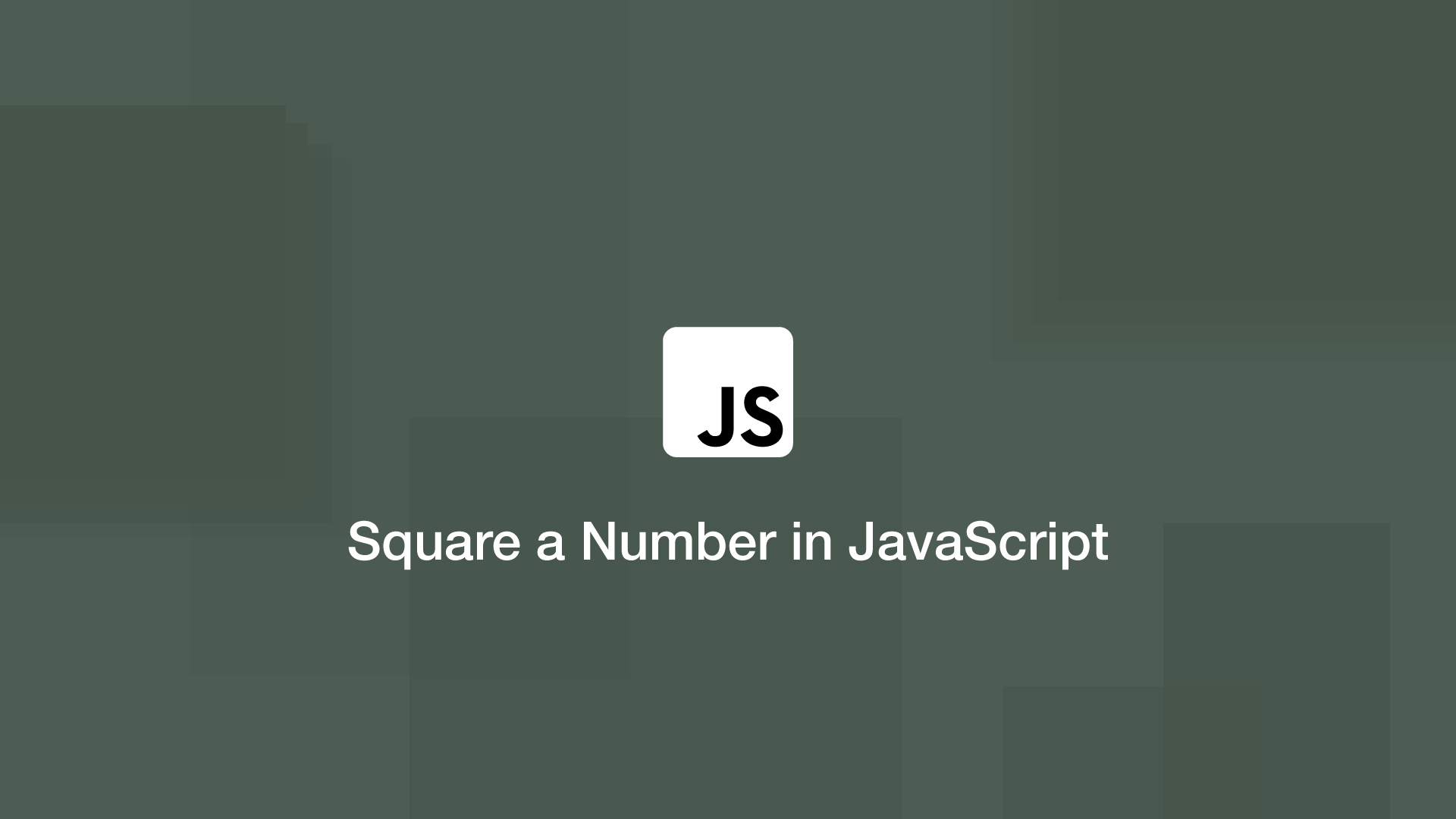# How to Square a Number in JavaScriptTo square a number in JavaScript use the `Math.pow()` function – this function takes two arguments, the first is the number to exponentiate and the second is the power to raise it by. To square a number we will use 2 as the second argument.

To demonstrate this, let's square 3, the formula of which is 3^2 = 9.

``````var result = Math.pow(3,2);

console.log(result);
``````
``````9
``````

## Square Numbers with the JavaScript Exponentiation Operator

In ECMAScript 6 JavaScript introduced the exponentiation operator which is `**` (two asterisks.) This will allow you to raise numbers to a power with a clear and simple syntax.

``````var result = 3 ** 2;

console.log(result);
``````
``````9
``````

## JavaScript Square Function

If you need to square multiple numbers within your program it might be worth creating a function that you can pass any number and get it back squared.

``````function squareNum(number) {
return number ** 2;
}

var result = squareNum(3);

console.log(result);
``````
``````9
``````

#### Related Tutorials### How to Create and Use Arrays in JavaScript

July 11, 2020### How to Use Maps in JavaScript

July 16, 2020### How to get Random Elements from an Array in JavaScript

September 13, 2020### How to Create and Use Lists (Arrays) in Python

September 01, 2020### How to Merge Strings or Arrays in JavaScript Using the concat() Method

September 09, 2020### How to use slice() & substring() to Get Parts of a String in JavaScript

September 12, 2020